Get instant live expert help with Excel or Google Sheets“My Excelchat expert helped me in less than 20 minutes, saving me what would have been 5 hours of work!”

#### Post your problem and you’ll get Expert help in seconds.

Your message must be at least 40 characters
Our professional Expert are available now. Your privacy is guaranteed.

# Sum by weekday

Excel allows us to sum the values based on the weekday by using SUMPRODUCT and WEEKDAY functions. This step by step tutorial will assist all levels of Excel users in summarizing the values based on the weekday.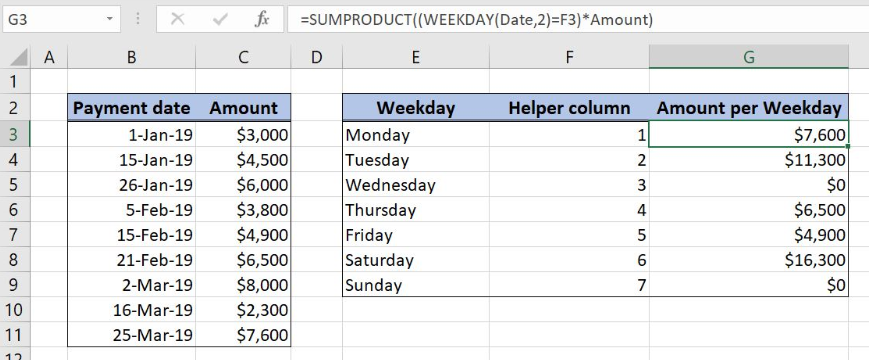Figure 1. Sum Amount by Weekday

## Syntax of the SUMPRODUCT Formula for Summing With Criteria

`=SUMPRODUCT(--(Criteria_range=Criteria)*Sum_Range)`

The parameters of the SUMPRODUCT function for summing with criteria are:

• Criteria_range – a cell range where we check if the criterion is met
• Criteria – a criterion for summing the data
• Sum_Range – a cell range to sum the data

## Syntax of the WEEKDAY Formula

`=WEEKDAY(date,[return_type])`

The parameters of the WEEKDAY function are:

• date – date value from which we want to get the weekday
• return_type – indicates the start day of the week (1- week starts from Sunday to Saturday, 2-weeks starts from Monday to Sunday)

## Setting up Our Data for Summing by Weekday

Our first table consists of 2 columns: “Payment date” (column B) and “Amount” (column C). The second one has three columns: “Weekday” (Column E), “Helper column” (Column F) and “Amount per Weekday” (Column G). The idea is to summarize the values from the column “Amount” based on the Weekday and to place the result in the column G.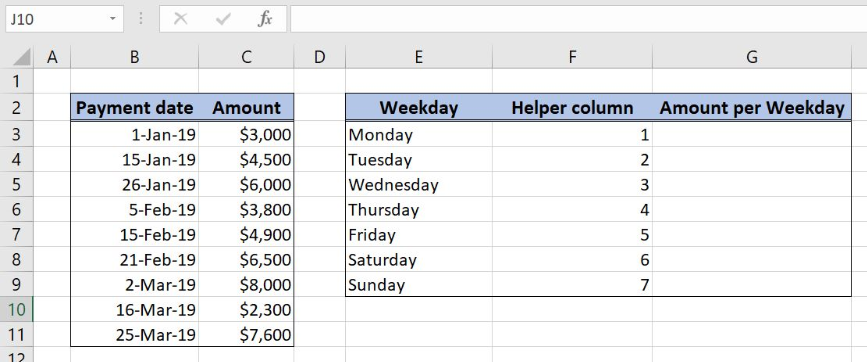Figure 2. Table structure for summarizing the “Amount” values by Weekday

## SUM by Weekday Using SUMPRODUCT and WEEKDAY Function

We want to get the total amount from column C based on the Weekday in column F. In order to make the formula more clear, we will create a named range Date for cell range B3:B12 and Amount for cell range C3:C11.

To create a named range we should follow the steps:

• Select the cell range that should be named
• Click on the name box in Excel
• Write the name for the cell range and press enter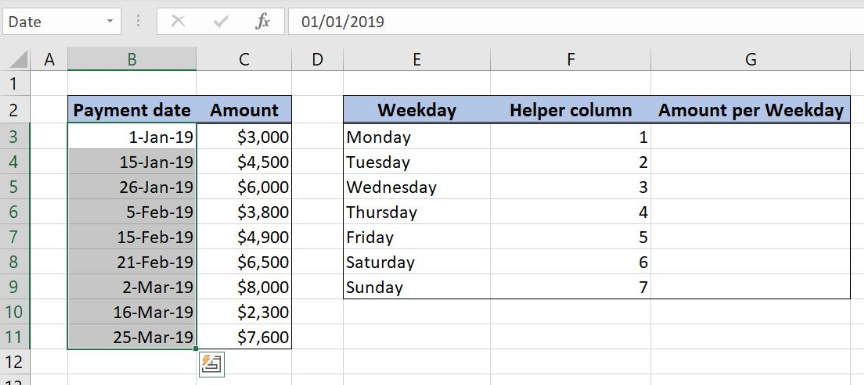Figure 3. Creating a named range Date for column “Payment date”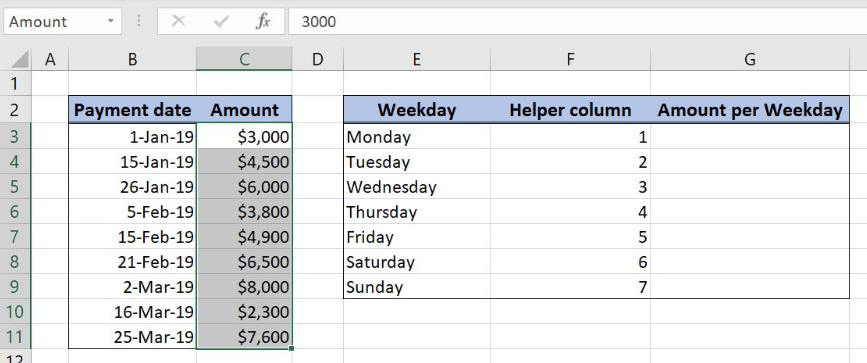Figure 4. Creating a named range Amount for column “Amount”

The formula for summarizing the values based on weekdays looks like:

`=SUMPRODUCT((WEEKDAY(Date,2)=F3)*Amount)`

The criteria_range in the SUMPRODUCT function is the formula part WEEKDAY(Date,2) while the criteria is the weekday number in the cell F3. Sum_range is named range Amount. In WEEKDAY function date is a named range Date, while the return_type is 2.

To apply the SUMPRODUCT function we need to follow these steps:

• Select cell G3 and click on it
• Insert the formula: `=SUMPRODUCT((WEEKDAY(Date,2)=F3)*Amount)`
• Press enter
• Drag the formula down to the other cells in the column by clicking and dragging the little “+” icon at the bottom-right of the cell.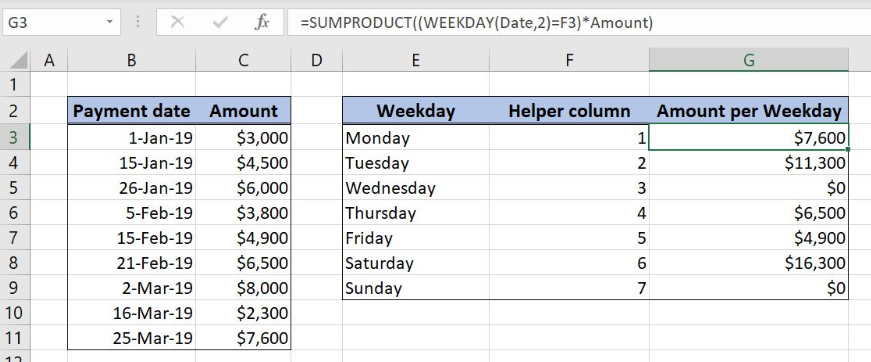Figure 5. Sum by Weekdays using SUMPRODUCT and WEEKDAY function

Formula WEEKDAY(Date,2 ) converts each date from the Date named range to the weekday number: `{2;2;6;2;5;4;6;6;1}`.

This array is then compared with the value 1 from the cell F3. Values in the “Helper column” represent the days in the week. Output array after comparison looks like: `{FALSE;FALSE;FALSE;FALSE;FALSE;FALSE;FALSE;FALSE;TRUE}`.

Array values from the WEEKDAY function are then multiplied with the values from the Amount named range creating a new array:

`{0;0;0;0;0;0;0;0;7600}`

The final result is the number 7600, a sum of all final array numbers.

Most of the time, the problem you will need to solve will be more complex than a simple application of a formula or function. If you want to save hours of research and frustration, try our live Excelchat service! Our Excel Experts are available 24/7 to answer any Excel question you may have. We guarantee a connection within 30 seconds and a customized solution within 20 minutes.

### Did this post not answer your question? Get a solution from connecting with the expert.Another blog reader asked this question today on Excelchat:
Solution examplesI need a formula to pop at the upper left corner of a spreadsheet. If I enter the month "January," I want the column number sum of January =SUM(AB11:AB75) from another section on the same excel page to pop right below the "January" cell, and not display the formula expression, but see the \$100.
Solved by T. Q. in 40 minsCan't add (SUM) in imported numbers from bank account
Solved by F. C. in 40 minsI need a formula to combine D2 to D100 to add together a column of numbers, then take away the same amount on the same row when column E is filled. i.e. column D is a price of an item, so the formula must calculate the total, then when the item is sold an 'a' is marked next to the item in column E, the formula then must deduct this amount from the total
Solved by X. W. in 20 minsI would like to have a diagram in a new sheet, where the horizontal axis is the days, as they are in column DX. Each day shall show the sum of all unique leads of that day, and I would like to be able to check via a box of checkboxes, which facilities are shown, the facilities are in column BC.
Solved by I. A. in 45 minsI am working on a cash flow projection. Part of the projection includes sales commissions. Our sales guys earn a monthly draw and then commission on sales after a certain amount. For example they may earn a monthly salary of 12,500 and earn additional commission after their commission equals \$150,000. What I need excel to do is sum a column if the values in the preceeding columns are greater than \$150,000. I've tried using the sumif and the if function in excel and it's not working correctly either way. Any suggestions?
Solved by G. W. in 19 mins## Subscribe to Excelchat.coAnother blog reader asked this question today on Excelchat: